﻿ Reliability Level of Pb-Sb-Cu Alloy Electrical Resistance Dependence on Its Melting Temperature and Copper Input Concentration

### Reliability Level of Pb-Sb-Cu Alloy Electrical Resistance Dependence on Its Melting Temperature and ...

C. I. Nwoye, A. O. Agbo, C. C. Nwogbu, S. Neife, E. M. AmehOPEN ACCESSPEER-REVIEWED

## Reliability Level of Pb-Sb-Cu Alloy Electrical Resistance Dependence on Its Melting Temperature and Copper Input Concentration

C. I. Nwoye1,, A. O. Agbo2, C. C. Nwogbu3, S. Neife4, E. M. Ameh2

1Department of Metallurgical and Materials Engineering, Nnamdi Azikiwe University, Awka, Nigeria

2Department of Metallurgical and Materials Engineering, Enugu State University of Science & Technology, Enugu, Nigeria

3Science Technical Vocation Schools Management Board, Enugu, Nigeria

4Department of Metallurgical and Materials Engineering, University of Nigeria Nsukka, Nigeria

### Abstract

This paper assesses the reliability level of Pb-Sb-Cu alloy electrical resistance dependence on its melting temperature and copper input concentration. The alloy was cast by pouring a stirred mixture of heated Pb-Sb alloy and powdered copper into a sand mould and then furnace cooled. Results of electrical test carried out indicate that the electrical resistance of the Pb-Sb-Cu alloy decreases with increase in the melting temperature of the Pb-Sb-Cu alloy. This invariably implied decrease in the electrical resistivity of the alloy. Increased copper addition (0.99-8.26 wt%) to the base alloy (Pb-Sb) also correspondingly decreased the electrical resistance. The experimental results were complement by results generated using a derived model. The validity of the two-factorial derived model expressed as: ξ = - 0.1248ɤ - 0.0398ϑ + 66.615 was rooted on the expression ξ - 66.615 = - 0.1248ɤ - 0.0398ϑ where both sides of the expression are correspondingly approximately equal. Statistical analysis of the experiment, derived model & regression model-predicted results shows that the standard errors incurred in predicting the Pb-Sb-Cu alloy electrical resistance for each value of the melting temperature and copper input were 0.1247, 0.1722 & 3.517 x 10-5 % and 0.4276, 0.1797 & 0.3593 % respectively. Evaluations indicate that Pb-Sb-Cu alloy electrical resistance per unit rise in the melting temperature and copper mass-input as obtained from experiment, derived model & regression model-predicted results were 0.2507, 0.2309 & 0.2496 Ω /°C and 0.094, 0.0866 & 0.0936 Ω / g respectively. Deviational analysis indicated that the maximum deviation of derived model-predicted electrical resistance from the experimental results was less than 3%. This translated into over 97% operational confidence and reliability level for the derived model and over 0.97 reliability coefficient for the Pb-Sb-Cu alloy electrical resistance dependence on the alloy melting temperature and copper input concentration.

### At a glance: Figures

12345
Prev Next

• Nwoye, C. I., et al. "Reliability Level of Pb-Sb-Cu Alloy Electrical Resistance Dependence on Its Melting Temperature and Copper Input Concentration." Materials Science and Metallurgy Engineering 1.2 (2013): 42-49.
• Nwoye, C. I. , Agbo, A. O. , Nwogbu, C. C. , Neife, S. , & Ameh, E. M. (2013). Reliability Level of Pb-Sb-Cu Alloy Electrical Resistance Dependence on Its Melting Temperature and Copper Input Concentration. Materials Science and Metallurgy Engineering, 1(2), 42-49.
• Nwoye, C. I., A. O. Agbo, C. C. Nwogbu, S. Neife, and E. M. Ameh. "Reliability Level of Pb-Sb-Cu Alloy Electrical Resistance Dependence on Its Melting Temperature and Copper Input Concentration." Materials Science and Metallurgy Engineering 1, no. 2 (2013): 42-49.

 Import into BibTeX Import into EndNote Import into RefMan Import into RefWorks

### 1. Introduction

Recently, the sterling usefulness of lead-antimony alloy has significantly prompted the need for intensive and extensive research and development aimed at expanding the applicability of the alloy in the areas of fabrication, production and corrosion resistance. Researches [1, 2, 3] have been carried out to improve the electrical conductivity of Pb-Sb alloy used as wet cell battery heads. Addition of cadmium to Pb-Sb was found  to enhance the electrical conductivity of Pb-Sb alloy tremendously. The scientist indicates that the produced Pb-Sb-Cd alloy cannot find application in battery heads and plates because Cd is very radioactive and is capable of causing volatile and explosive reaction when in contact with sulphuric acid for a long time. Report  has shown that addition of silver to Pb-Sb alloy increases very significantly the electrical conductivity of the alloy. The researchers opined that the formed alloy contains impurities such as Au, As, Sn, Cu and S which creates unstable electric field in the alloy of Pb-Sb-Ag and invariably, unstable current reading. The researchers concluded that the alloy cannot be used for battery heads and plates since it obscures the precise electromotive force of the electrolyte in the battery.

Addition of copper powder by dispersion to Pb-Sb alloy has been discovered  to improve the electrical conductivity of alloy greatly. It is believed that this breakthrough was possible because Cu used, had high purity level (99.8%).

Studies  have shown that the higher the temperature applied to metals and alloys, the greater the bonds broken, and the higher the level of conductivity attained. The scientist indicates that the resistivity of engineering materials such as metals and alloys depends on the temperature at which the material is exposed. The researcher concluded that increase in the temperature of materials reduces its resistivity. The report  indicates that the energy gap; minimum additional energy which a bonding electron must acquire to leave the bond and, hence become free to conduct electricity decreases with increasing temperature and impurity content. The report also revealed that the resistivity increases with increase in the energy gap.

Tin-antimony-copper alloys was produced and studied  to assess its applicability in the electronics and telecommunications industry. Results of the investigation revealed that the alloy can be used as favourable solders for consumer electronics. The electrical conductivity and viscosity studies were carried out in a wide temperature range above the liquidus. A melting-solidification region was investigated by Differential Scanning Calorimetry (DSC) and scaling relations were proposed.

The aim of this work is to assess the reliability level of Pb-Sb-Cu alloy electrical resistance dependence on its melting temperature and copper input concentration.

### 2. Materials and Methods

Alloy preparation

The materials used are antimonial lead scraps and electrolytic copper powder of grain size < 425μm. The collected antimonial lead were first of all, melted together (at the forge) in order to obtain a fairly uniform composition of the alloy. The mild steel melting crucible used was of dimension 260mm long, 200mm wide and 100mm breadth.

Mould preparation

The mould was prepared by first sieving the sand for aeration and mixing with 6% moisture to give good green strength. The mould box used was made from cast metal frame and has the dimension: 500 mm long, 300mm wide and 100mm breadth. Long hollow cylindrical pipes (with locks) of 85mm long and 9mm diameter were used as patterns for the cast. The mould was allowed to dry prior to casting.

Casting and cooling

Various quantities of lead antimony alloy (in wt %) and Cu powder was in separate cases placed on the crucible which was then placed inside the furnace. The alloys were cast by pouring a stirred mixture of Cu powder and Pb-Sb alloy heated to 425°C, into the locked cylindrical patterns inserted into the sand mould. The furnace was switched off and the patterns were unlocked to release the cast alloys which were then collected in another crucible using a steel tong. The crucible containing the cast alloys were then place in the furnace and allowed to cool down through the furnace temperature. The control alloys were cast by just pouring only the molten Pb-Sb into the pattern inserted into the sand mould and also cooled as in the case of Pb-Sb-Cu alloy.

Heat treatment

The cast alloys were cut to lengths: 0.075m before being heat treated at a temperature of 180°C for 1 hr to relieve stresses incurred during solidification of the alloys. The heat treatment was carried out to also homogenize the microstructure of the alloys prior to the impact testing process.

Electrical measurements

Following the heat treatment process, electric current I, flowing through the alloy was measured and other electrical properties associated with current flow calculated using appropriate equations. The electromotive force from the dry battery equals 2.9V, being voltage supply to the alloys. The tested specimen were thereafter melted and their melting temperatures recorded correspondingly against their respective values of electric current, resistance, resistivity and conductivity.

Calculation of electrical parameters

According to Ohm’s law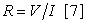(1)

Where

R = Resistance of the alloy (Ω)

V = Voltage supply to the alloy (V)

I = Current flowing through the alloy (A)

The resistance of the Pb-Sb-Cu alloy was calculated using equation (1). Also, the resistivity of the alloy was calculated using the equation;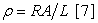(2)

Where

ρ = Resistivity of the alloy (Ωm)

L = 0.075(Length of the alloy material) (m)

A = 6.364 x 10-5 (Cross sectional area of the alloy (m2)

The cross sectional area of the alloy material was calculated using the equation;(3)

Where

Π = 22/7 Substituting these values into

D = 0.9cm; (Diameter of cross- section of the sample)

### 3. Results and Discussions

Results of chemical analysis carried out on the materials used (as shown in Table 1) indicate that antimonial lead contains about 3.3% Cu in addition to Pb and Sb present. The percentage composition of the powdered Cu used was as received.

#### Table 2. Electrical properties of Pb-Sb alloy cooled in furnace (Alloy control of melting temperature 420°C)

Table 2 shows the electrical properties of furnace cooled Pb-Sb alloy (control). This alloy melted at a temperature of 420°C.

Increasing the temperature of metals and alloys reduces their respective resistivities . Substitution of equation (1) into (2) gave ρ = V A/L I which was also the Pb-Sb-Cu alloy resistivity, ρ. Table 3 indicated that increase in the alloy melting temperature resulted to decrease in the resistivity of the alloys in accordance with previous report . The decreased alloy electrical resistivity in turn resulted to increase in the current flowing through the alloy. This was sequel to the fact that increase in the temperature of metals and alloys excites the electron and increases the number of bonds broken, hence increasing flow of electron through increased vibrational motion into the conduction band .

#### Table 3. Variation of copper addition (to Pb-Sb alloy) and melting temperature of Pb-Sb-Cu alloy on the electrical current flow, resistance and resistivity of the alloy formed

The electrical conductivity α of the Pb-Sb-Cu alloy was calculated from equation (2) as the reciprocal of the electrical resistivity ρ. Table 3 also shows that increase in copper addition (up to 8.26 wt %) to the base alloy (Pb-Sb) not only increased correspondingly the current flow, but the electrical conductivity and in turn decreased correspondingly the electrical resistance and resistivity of Pb-Sb-Cu alloy so produced. This was attributed to the behaviour of Cu (added to the base alloy (Pb-Sb)) as impurity atoms. These impurity atoms were strongly believed to have reduced the electrical resistivity of the Pb-Sb-Cu alloy in agreement with past report , and invariably increased the current flow.

3.1. Model Formulation

A computational analysis of experimental results in Table 3 was carried out using a software; C-NIKBRAN . The software proffered an empirical core expression using results of the analysis. The expression indicates that;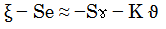(4)

Introducing the values of Se, S and K into equation (4) reduces it to: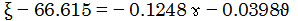(5)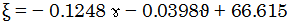(6)

Where

(ξ) = Pb-Sb-Cu electrical resistance Pb (Ω)

(ɤ) = Melting temperature (°C)

(ϑ) = Mass-input of copper ( g)

Se = 66.615, S = 0.1248 and K = 0.0398: empirical constants determined using C- NIKBRAN .

Equation (6) is the derived model.

3.2. Boundary and Initial Condition

Consider Pb-Sb alloy (in a furnace) garnished with copper powder. The furnace atmosphere was not contaminated i.e (free of unwanted gases and dusts). Initially, atmospheric levels of oxygen are assumed just before adding copper to Pb-Sb alloy (due to air in the furnace). Length of cast alloys: 0.075m, Pb-Sb-Cu alloy melting temperature range: 425 - 440oC, heat treatment temperature: 180°C.

The boundary conditions are: furnace oxygen atmosphere at the top and bottom of the melt interacting with the gas phase. At the bottom of the particles, a zero gradient for the gas scalar are assumed and also for the gas phase at the top of the particles. The sides of the particles are taken to be symmetries.

3.3. Model Validity

The validity of the model was strongly rooted in the core model equation (equation (5)) where both sides of the equation are correspondingly approximately equal.

#### Table 4. Variation of ξ - 66.615 with - 0.1248ɤ - 0.0398ϑ

Table 4 also agrees with equation (5) following the values of ξ- 66.615 and - 0.1248ɤ - 0.0398 computed from the experimental results in Table 3.

Furthermore, the derived model was validated by comparing the Pb-Sb-Cu alloy electrical resistance evaluated from experimental and derived model. This was done using various evaluative techniques such as statistical, graphical, computational and deviational analysis.

3.4. Statistical Analysis

Standard Error (STEYX)

The standard errors in predicting the Pb-Sb-Cu alloy electrical resistance for each value of the alloy melting temperature and copper input concentration as obtained from experiments & derived model-predicted results were 0.1247 & 0.1722 % and 0.4276 & 0.1797 % respectively.

Figure 1. Coefficient of determination between Pb-Sb-Cu alloy electrical resistance and melting temperature as obtained from experiment
Figure 2. Coefficient of determination between Pb-Sb-Cu alloy electrical resistance and melting temperature as predicted by model
Figure 3. Coefficient of determination between Pb-Sb-Cu alloy electrical resistance and copper input concentration as obtained from experiment
Figure 4. Coefficient of determination between Pb-Sb-Cu alloy electrical resistance and copper input concentration as predicted by model
Figure 5. Comparison of Pb-Sb-Cu alloy electrical resistances (relative to melting temperature) as obtained from experiment and derived model
Figure 6. Comparison of Pb-Sb-Cu alloy electrical resistances (relative to copper input concentration) as obtained from experiment and derived model
Figure 7. Comparison of Pb-Sb-Cu alloy electrical resistances (relative to melting temperature) as obtained from experiment, derived model and regression model
Figure 8. Comparison of Pb-Sb-Cu alloy electrical resistances (relative to copper input concentration) as obtained from experiment, derived model and regression model

Correlation (CORREL)

The correlation coefficients between Pb-Sb-Cu alloy electrical resistance and alloy melting temperature as well as copper input concentration were calculated from the results of derived model and experiment. This was done by considering the coefficients of determination R2 from Figure 1 - Figure 4 and then evaluating them using the expression: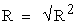(7)

The evaluated correlations (using Microsoft Excel version 2003) are shown in Table 5 and Table 6. These evaluated results indicate that the derived model predictions are significantly reliable, considering the proximate agreement between actual experimental and model-predicted results.

#### Table 6. Comparison of the correlations evaluated from derived model predicted and ExD results based on copper input concentration

3.6. Graphical Analysis

Comparative graphical analysis of Figure 5 and Figure 6 shows extremely close alignment of the curves from the experimental (ExD) and model-predicted (MoD) Pb-Sb-Cu alloy electrical resistance. Furthermore, the degree of alignment of these curves is indicative of the proximate agreement between both experimental and the model-predicted alloy electrical resistance.

### 4. Comparison of Derived Model with Standard Model

The validity of the derived model was further verified through application of the regression model (Reg) (Least Square Method using Excel version 2003) in predicting the trend of the experimental results. Comparative analysis of Figure 7 and Figure 8 show very close alignment of curves which depicted significantly similar trend of data point’s distribution for experimental (ExD), derived model (MoD) and regression model-predicted (ReG) results of the Pb-Sb-Cu alloy electrical resistance. Moreover, the evaluated correlations between the alloy electrical resistance and its melting temperature & copper input concentration for regression model-predicted results gave 1.0000 & 0.9669 respectively. These values are in proximate agreement with both experimental and derived model-predicted results. The standard errors incurred in predicting the alloy electrical resistance for each value of the melting temperature and copper input concentration, as evaluated from regression model-predicted results were 3.517 x 10-5 and 0.3593 % respectively.

4.1. Computational Analysis

Comparative analyses of the Pb-Sb-Cu alloy electrical resistance evaluated from experimental, derived model-predicted and regression model predicted results were carried out to ascertain the degree of validity of the derived model. This was done by comparing evaluated results of the alloy electrical resistance per unit rise in melting temperature and per unit copper input concentration.

Alloy electrical resistance per unit copper input concentration

Alloy electrical resistance per unit copper input concentration ξ / ϑ (Ω/ g) was calculated from the equation;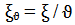(8)

Re-written as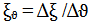(9)

Equation (9) is detailed as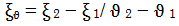(10)

Where

Δξ = Change in the extracted lead concentration ξ2, ξ 1 at leaching times ϑ 2 , ϑ 1.

Considering the points (425, 13.49) & (440, 9.73), (425, 13.376) & (440,9.912) and (425,13.537) & (440, 9.7929) as shown in Figure 7, and designating them as (ϑ1, ξ1) & (ϑ2, ξ2) for experimental, derived model and regression model predicted results respectively, and then substituting them into equation (10), gives the slopes: - 0.0940, - 0.0866 & - 0.0936 Ω/ g respectively as their corresponding alloy electrical resistance per unit copper input concentration.

Alloy electrical resistance per unit rise in melting temperature

Similarly, substituting into equation (10) points (425, 13.49) & (440, 9.73), (425, 13.376) & (440,9.912) and (425,13.537) & (440, 9.7929) culled from Table 3 and Figure 8, as (ɤ1, ξ1) & (ɤ2, ξ2) for experimental, derived model and regression model predicted results respectively also gives the slopes: - 0.2507, - 0.2309 & - 0.2496 Ω/°C respectively as their corresponding alloy electrical resistance per unit rise in the melting temperature. The proximity between values in each result set indicates significantly high validity level for the derived model.

It is very pertinent to state that the actual alloy electrical resistance per unit copper input concentration and rise in the melting temperature (as obtained from experiment, derived model and regression model) was just the magnitude of the signed value. The associated sign preceding these values signifies that the associated slope tilted to negative plane. Based on the foregoing, the Pb-Sb-Cu alloy electrical resistance per unit copper input concentration and rise in the melting temperature as obtained from experiment, derived model & regression model were 0.0940, 0.0866 & 0.0936 Ω/ g and 0.2507, 0.2309 & 0.2496 Ω/°C respectively.

4.2. Deviational Analysis

Critical Comparative analysis of extracted lead concentrations obtained from experiment and derived model show deviations on the part of the model-predicted values relative to values obtained from the experiment. This is attributed to the fact that the surface properties of the ore and the physiochemical interactions between the ore and bioleaching solution, which played vital roles during the extraction process, were not considered during the model formulation. This invariably necessitated the introduction of correction factor, to bring the model-predicted extracted lead concentration to those of the corresponding experimental values.

The deviation Dv, of model-predicted alloy electrical resistance from the corresponding experimental result was given by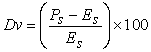(11)
Figure 9. Variation of derived model predicted electrical resistance with melting temperature relative to its associated deviation from experimental results
Figure 10. Variation of deviation (of derived model predicted electrical resistance from experimental result) with the alloy melting temperature and copper input concentration

Figure 9 and Figure 10 show that the maximum deviation of the model-predicted Pb-Sb-Cu alloy electrical resistance from the corresponding experimental values was less than 3% and quite within the acceptable deviation limit of experimental results. Consideration of equation (11) and critical analysis of Table 7, Figure 1-Figure 4, Figure 9 and Figure 10 show that the least and highest magnitudes of deviation of the model-predicted alloy electrical resistance (from the corresponding experimental values) are - 0.42 and 2.98%.This corresponds to Pb-Sb-Cu alloy electrical resistances: 10.934 and 12.553 Ω; alloy melting temperatures:435 and 430°C as well as copper input concentrations: 35g (6.54 wt %) and 10g (1.96 wt%) respectively.

#### Table 7. Variation of correction factor (to model-predicted alloy electrical resistance) with alloy melting temperature and copper input concentration

Correction factor, Cf to the model-predicted results was given by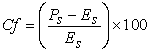(12)

Where

Es and Ps are Pb-Sb-Cu alloy electrical resistance evaluated from experiment and derived model respectively.

Equations (11) and (12) indicate that correction factor is the negative of the deviation.

The correction factor took care of the negligence of operational contributions of surface properties of the added copper powder and the physiochemical interactions between the copper powder and Pb-Sb matrix (control), which actually played vital role during the Pb-Sb-Cu alloy production. The model predicted results deviated from those of the experiment because these contributions were not considered during the model formulation. Introduction of the corresponding values of Cf from equation (12) into the model gives exactly the corresponding experimental values of the alloy electrical resistance.

On the other hand, consideration of equation (12) and critical analysis of Table 7 indicates that the evaluated correction factors are negative of the deviation as shown in equations (11) and (12). Table 7, Figure 1-Figure 4, Figure 9 and Figure 10 show that the least and highest magnitudes of correction factor to the model-predicted alloy electrical resistance are 0.42 and - 2.98%.This also corresponds to Pb-Sb-Cu alloy electrical resistances: 10.934 and 12.553 Ω; alloy melting temperatures: 435 and 430°C as well as copper input concentrations: 35 (6.54wt %) and 10g (1.96 wt %) respectively.

It is important to state that the deviation of model predicted results from that of the experiment is just the magnitude of the value. The associated sign preceding the value signifies that the deviation is a deficit (negative sign) or surplus (positive sign).

### 5. Effect of Copper Addition on the Pb-Sb Alloy Microstructure

Critical comparative analysis of Figure 11-Figure 15 shows a very significant and conspicuous variation between the microstructure of Pb-Sb alloy (Figure 11) which is the control and the others. These Figures show that the microstructure of Pb-Sb alloy changes with addition of various quantities of copper. It is strongly believed that the various levels of electrical conductivities of produced Pb-Sb-Cu alloy were brought about by the corresponding microstructural modification resulting from the respective copper addition. Table 3 shows clearly that the electrical conductivity of Pb-Sb-Cu alloy increases with copper addition.

### 6. Conclusion

Assessment of the reliability level of Pb-Sb-Cu alloy electrical resistance dependence on its melting temperature and quantified copper input was carried out. The electrical resistance of the Pb-Sb-Cu alloy decreases with increase in the melting temperature of the Pb-Sb-Cu alloy and mass-input of the copper. The validity of the two-factorial derived analytical model was rooted on the expression ξ - 66.615 = - 0.1248ɤ - 0.0398ϑ where both sides of the expression are correspondingly approximately equal. The Pb-Sb-Cu alloy electrical resistance per unit rise in the melting temperature and copper mass-input as obtained from experiment, derived model & regression model-predicted results were 0.2507, 0.2309 & 0.2496 Ω /°C and 0.094, 0.0866 & 0.0936 Ω / g respectively. The maximum deviation of derived model-predicted electrical resistance from the experimental results was less than 3%. This translates into over 97% operational confidence and reliability level for the derived model and over 0.97 reliability coefficient for the Pb-Sb-Cu alloy electrical resistance dependence on the alloy melting temperature and quantified copper input.

### References

  Blumenthal B. (1944). Effects of Silver on the Electrical Conductivity of Lead-Antimony Alloy, Trans. Amer. Inst. Min. Met, England. 149-156.In article  Rollason, E. C., and Hysel, V. B. (1940). Effect of Cadmium on the Electrical Conductivity of Lead-Antimony Alloy, J. Inst. Metals, London. 59-66.In article  Nwoye, C. I. (2000). Effect of Copper Powder Dispersion on the Electrical Conductivity of Lead-Antimony Alloy, M. Eng. Thesis, Nnamdi Azikiwe University, Awka.In article  Ijomah, M. N. C. (1992).The Structure and Properties of Engineering Materials, Christon Publishers, Awka. pp 56-58.In article  Plevachuk,Yu., Sklyarchuk,V., Yakymovych, A., Svec, P., Janickovic, D. and Illekova, E. (2011). Electrical conductivity and viscosity of liquid Sn-Sb-Cu alloys. Journal of Materials Science: Materials in Electronics; 22(6):631In article CrossRef  www.ndt-ed.org/General Resources/Material Properties.In article  Okeke, P. C. (1987). Introduction to Physics, Gerek Publishers, Enugu. pp 85-93.In article  Nwoye, C. I. (2008). C-NIKBRAN; Data Analytical Memory.In article